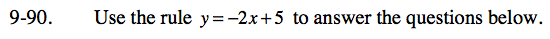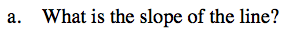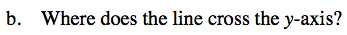### Home > CC3 > Chapter Ch9 > Lesson 9.2.3 > Problem9-90

9-90.
1. Use the rule y = –2x + 5 to answer the questions below. 9-90 HW eTool (Desmos). Homework Help ✎

1. What is the slope of the line?

2. Where does the line cross the y-axis?Pick two points on the line and find the change in y-values over the change in x-values to find the slope.
What do you get? Does this value correspond with a value in the rule above?Where on the graph does the line cross the y-axis?
Also, logically, the graph will cross the y-axis when x = 0.
Using the rule, can you calculate this value?
What do you get? Does this value correspond with a value in the rule above?

(0, 5)